# Getting started with OpenACC and Nvidia Nsight

OpenACC is a user-driven directive-based performance-portable parallel programming model. From the OpenACC homepage.

In many ways OpenACC is similar to OpenMP, but with a focus on running the code on accelerators (such as GPUs). OpenACC defines a set of directives (for both `C/C++` and `Fortran`) that can be included in existing code to transition the runtime to accelerators.

Accelerators, like the Nvidia GPUs on Saga, are great for numerical calculations and applications that work on the “SIMD” - Single Instruction Multiple Data principle, (where one or more operations are applied to a large number of datapoints independently of each other). Examples include operations like `gemm` which can be 6 times faster than on the CPU, or generating random numbers which can be 70 times faster!

Note

If you know some OpenACC or want to see tips for larger applications take a look at the tip section at the bottom.

Note

We have also included a Fortran example at the end of this document.

Tip

For a summary of available directives we have used this reference guide.

## Introduction

This guide will introduce the concept of OpenACC directives in `C/C++` code, how to compile and run such programs on Saga and how to use Nvidia Nsight to profile and optimize code.

After reading this guide you should:

• Know what OpenACC is

• Know how to compile `C/C++` OpenACC programs on Saga

• Know how to run OpenACC programs on GPUs on Saga

• Know how to run OpenACC programs with a profiler (`nsys`) on Saga

• Know how to understand the basic Nsight user interface

• Know how to optimize OpenACC programs based on profiler results

## OpenACC

To begin we will need an example program that does some calculations that we would like to speed up.

We have selected an example based on heat dissipation utilizing Jacobi iterations. The initial source can be found in `jacobi_serial.c`, shown below:

```/**
* Serial implementation of the Jacobi iteration
*/

#include <math.h>
#include <stdio.h>
#include <stdlib.h>

// Number of rows and columns in our matrix
static const int NUM_ELEMENTS = 2000;
// Maximum number of iterations before quiting
static const int MAX_ITER = 10000;
// Error tolerance for iteration
static const float MAX_ERROR = 0.01;
// Seed for random number generator
static const int SEED = 12345;

int main (int argc, char** argv) {
// Initialize random number generator
srand (SEED);
// Create array to calculate on
float array[NUM_ELEMENTS][NUM_ELEMENTS];
// Fill array with data
for (int i = 0; i < NUM_ELEMENTS; i++) {
for (int j = 0; j < NUM_ELEMENTS; j++) {
// The following will create random values between [0, 1]
array[i][j] = (float) rand () / (float) RAND_MAX;
}
}
// Before starting calculation we will define a few helper variables
float arr_new[NUM_ELEMENTS][NUM_ELEMENTS];
float error = __FLT_MAX__;
int iterations = 0;
// Perform Jacobi iterations until we either have low enough error or too
// many iterations
while (error > MAX_ERROR && iterations < MAX_ITER) {
error = 0.;
// For each element take the average of the surrounding elements
for (int i = 1; i < NUM_ELEMENTS - 1; i++) {
for (int j = 1; j < NUM_ELEMENTS - 1; j++) {
arr_new[i][j] = 0.25 * (array[i][j + 1] +
array[i][j - 1] +
array[i - 1][j] +
array[i + 1][j]);
error = fmaxf (error, fabsf (arr_new[i][j] - array[i][j]));
}
}
// Transfer new array to old
for (int i = 1; i < NUM_ELEMENTS - 1; i++) {
for (int j = 1; j < NUM_ELEMENTS - 1; j++) {
array[i][j] = arr_new[i][j];
}
}
iterations += 1;
}
return EXIT_SUCCESS;
}
```

`jacobi_serial.c`

### Compiling and running on Saga

To compile this initial version on Saga we will need to load the `Nvidia HPC SDK`. This can be done with the following command:

```\$ module load NVHPC/20.7
```

Note

You can check if a newer version of `NVHPC` is available by issuing the command `module avail NVHPC`

Then to compile or serial version we will invoke the `nvc` compiler with the following command:

```\$ nvc -g -fast -o jacobi jacobi_serial.c
```

We can run this program on a compute node by issuing the following:

```# Run on compute node with 512MB of memory for a maximum of 2 minutes
\$ srun --account=<your project number> --time=02:00 --mem-per-cpu=512M time ./jacobi
# The first number outputted should be the number of seconds it took to run the
# program:
# 40.79user 0.01system 0:40.91elapsed 99%CPU (0avgtext+0avgdata 35212maxresident)k
# 5144inputs+0outputs (18major+1174minor)pagefaults 0swaps
```

### Initial transition

To begin transitioning the code to run on a GPU we will insert the `kernels` directive into the code. The `kernels` directive tells OpenACC that we would like everything inside the directive to be run on the GPU, but it is up to the compiler to decide how to best do this.

It is always a good idea to begin with the `kernels` directive as that is the easiest and it gives the compiler a lot of flexibility when translating the code. `kernels` is also a good way to understand if the compiler is not able to optimize something and if we need to rewrite some code to better run on the GPU.

The code is available in `jacobi_kernels.c` and the changes applied are shown below.

```  while (error > MAX_ERROR && iterations < MAX_ITER) {
error = 0.;
#pragma acc kernels
{
// For each element take the average of the surrounding elements
for (int i = 1; i < NUM_ELEMENTS - 1; i++) {
for (int j = 1; j < NUM_ELEMENTS - 1; j++) {
arr_new[i][j] = 0.25 * (array[i][j + 1] +
array[i][j - 1] +
array[i - 1][j] +
array[i + 1][j]);
error = fmaxf (error, fabsf (arr_new[i][j] - array[i][j]));
}
}
// Transfer new array to old
for (int i = 1; i < NUM_ELEMENTS - 1; i++) {
for (int j = 1; j < NUM_ELEMENTS - 1; j++) {
array[i][j] = arr_new[i][j];
}
}
}
iterations += 1;
}
```

`jacobi_kernels.c`

As can be seen in the code above we have added the `kernels` directive around the main computation that we would like to accelerate.

To compile the above we need to tell `nvc` that we would like to accelerate it on GPUs. This can be done with the `-acc` flag. We will also add the `-Minfo=accel` flag which informs the compiler that we would like it to inform us of what it is doing with accelerated regions. The full command is as follows.

```\$ nvc -g -fast -acc -Minfo=accel -o jacobi jacobi_kernels.c
```

When running this command pay special attention to the information it is telling us about the accelerated region.

```main:
40, Generating implicit copyin(array[:][:]) [if not already present]
Generating implicit copyout(array[1:1998][1:1998]) [if not already present]
Generating implicit copy(error) [if not already present]
Generating implicit copyout(arr_new[1:1998][1:1998]) [if not already present]
42, Loop is parallelizable
43, Loop is parallelizable
Generating Tesla code
42, #pragma acc loop gang, vector(4) /* blockIdx.y threadIdx.y */
Generating implicit reduction(max:error)
43, #pragma acc loop gang, vector(32) /* blockIdx.x threadIdx.x */
52, Loop is parallelizable
53, Loop is parallelizable
Generating Tesla code
52, #pragma acc loop gang, vector(4) /* blockIdx.y threadIdx.y */
53, #pragma acc loop gang, vector(32) /* blockIdx.x threadIdx.x */
```

In the above output the numbers corresponds to line numbers in our `jacobi_kernels.c` source file and the comments show what `nvc` intends to do with each line.

Before we start profiling to see what we can optimize, lets run the program to learn the additional `Slurm` parameters needed for running with GPU on Saga. The following is the new command needed (notice the added `--partition=accel` and `--gpus=1` flags)

```\$ srun --account=<your project number> --time=02:00 --mem-per-cpu=512M --partition=accel --gpus=1 time ./jacobi
```

`--partition=accel` is needed to tell `Slurm` to only run on nodes on Saga with GPUs and the `--gpus=N` line tells `Slurm` that we would like to have access to `N` GPUs (`accel` nodes on Saga have `4` separate GPUs, above we are asking for only one GPU).

### Profiling

To profile the `kernels` version of our program we will here transition to Job Scripts. This will make it a bit easier to make changes to how the program is run and also makes it a bit more reproducible.

The `Slurm` script is available as `kernels.job` and is show below.

```#!/bin/sh

#SBATCH --job-name=openacc_guide_kernels
#SBATCH --time=05:00
#SBATCH --mem-per-cpu=512M
#SBATCH --partition=accel
#SBATCH --gpus=1

set -o errexit  # Exit the script on any error
set -o nounset  # Treat any unset variables as an error

module --quiet purge  # Reset the modules to the system default
module list  # List modules for easier debugging

# Run the program through the Nsight command line profiler 'nsys'
# The '-t' flag tells the profiler which aspects it should profile, e.g. CUDA
# and OpenACC code
# The '-f' flag tells the profiler that it can override existing output files
# The '-o' flag tells the profiler the name we would like for the output file
nsys profile -t cuda,openacc -f true -o kernels ./jacobi
```

`kernels.job`

Run this script by issuing

```\$ sbatch kernels.job
```

The end result should be a file called `kernels.qdrep` which contains the profiling information. Download this file to your local computer to continue with this guide.

`kernels.qdrep`

## Nsight

We will continue this guide `kernels.qdrep` as the profiling result to view.

Note

To view images in a larger format, right click and select `View Image`

To begin, start Nsight Systems on your own machine, giving the following view.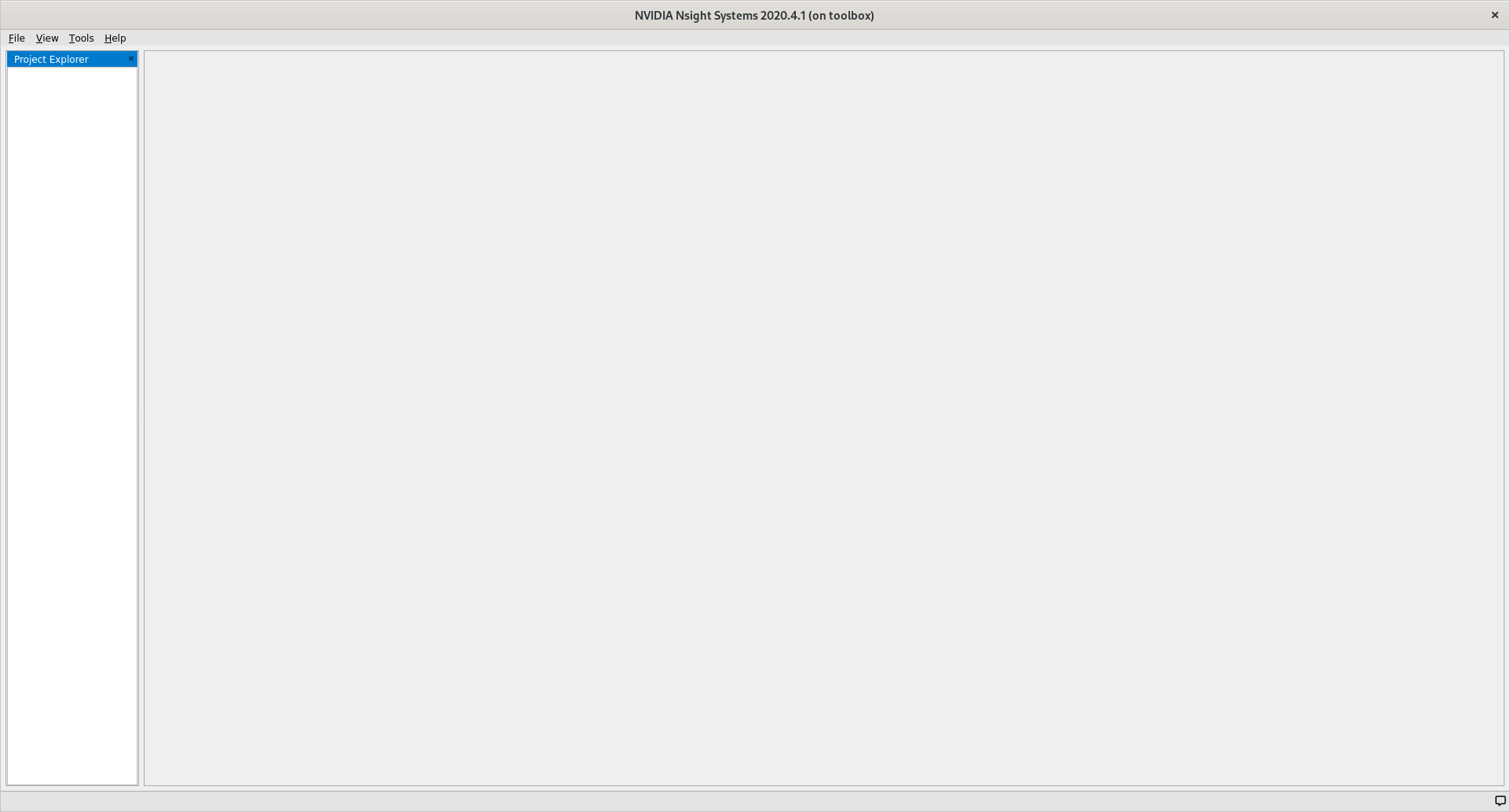To open our profiling result click `File`, then `Open` and navigate to the folder where you stored `kernels.qdrep`. Loading this file should give you the following view.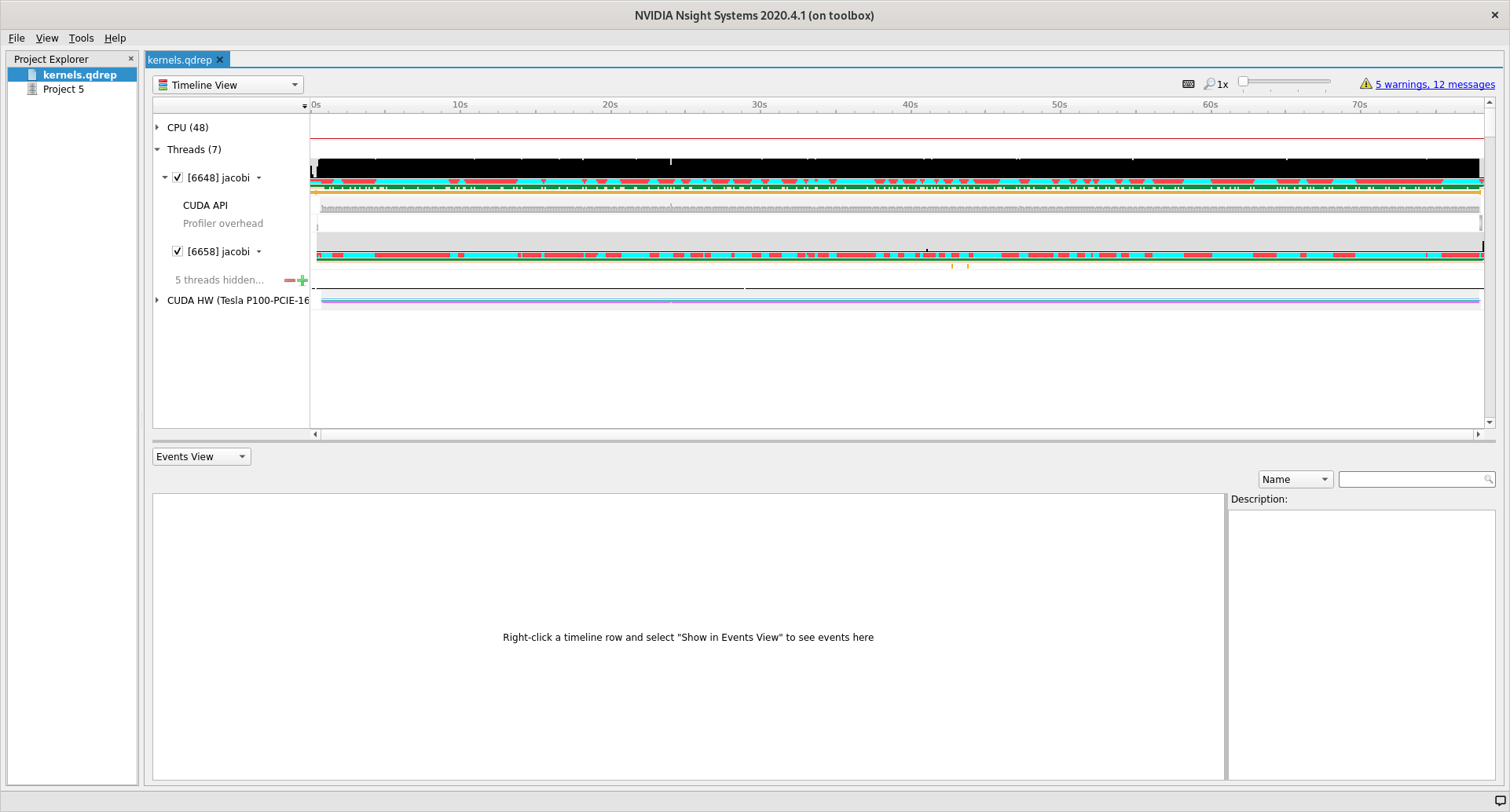### User interface

The user interface of Nsight is comprised of three main areas and two drop down menus that control what is shown in the different areas.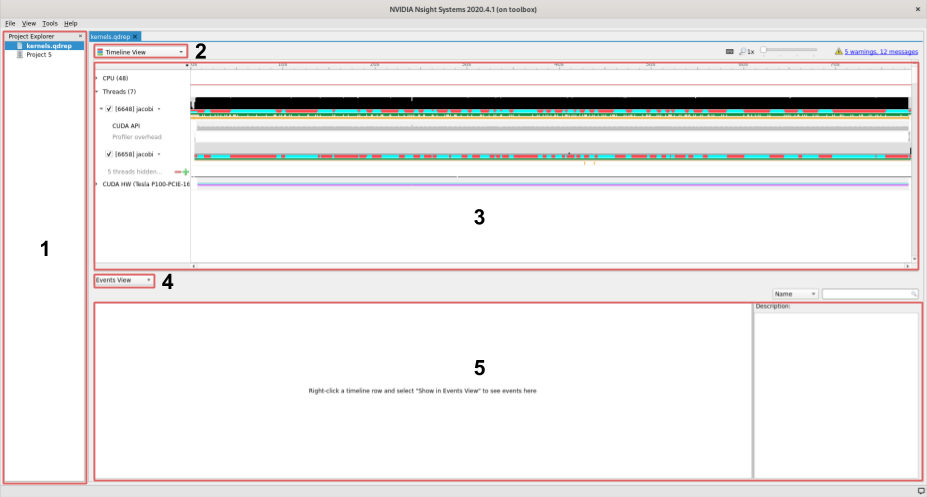1. On the left we find the project area, this list shows your project and profiles that you have loaded.

2. The left topmost dropdown menu selects which view to show

3. In the middle of the user interface we find the main view, currently showing the timeline of our profile. This view changes depending on the choice made in the dropdown menu marked with a `2`.

4. The second dropdown, in the middle of the screen, selects different views for the bottommost area.

5. The area at the bottom shows additional information about the profile together with the timeline view.

### Views

Using the topmost dropdown menu, marked with `2` in the picture above, we can select different views for the current profile.

When first opening a new profile it can be informative to start with the `Diagnostics Summary`. This view shows a summary of the profile and can give great hints about what went wrong if the profile is not as expected.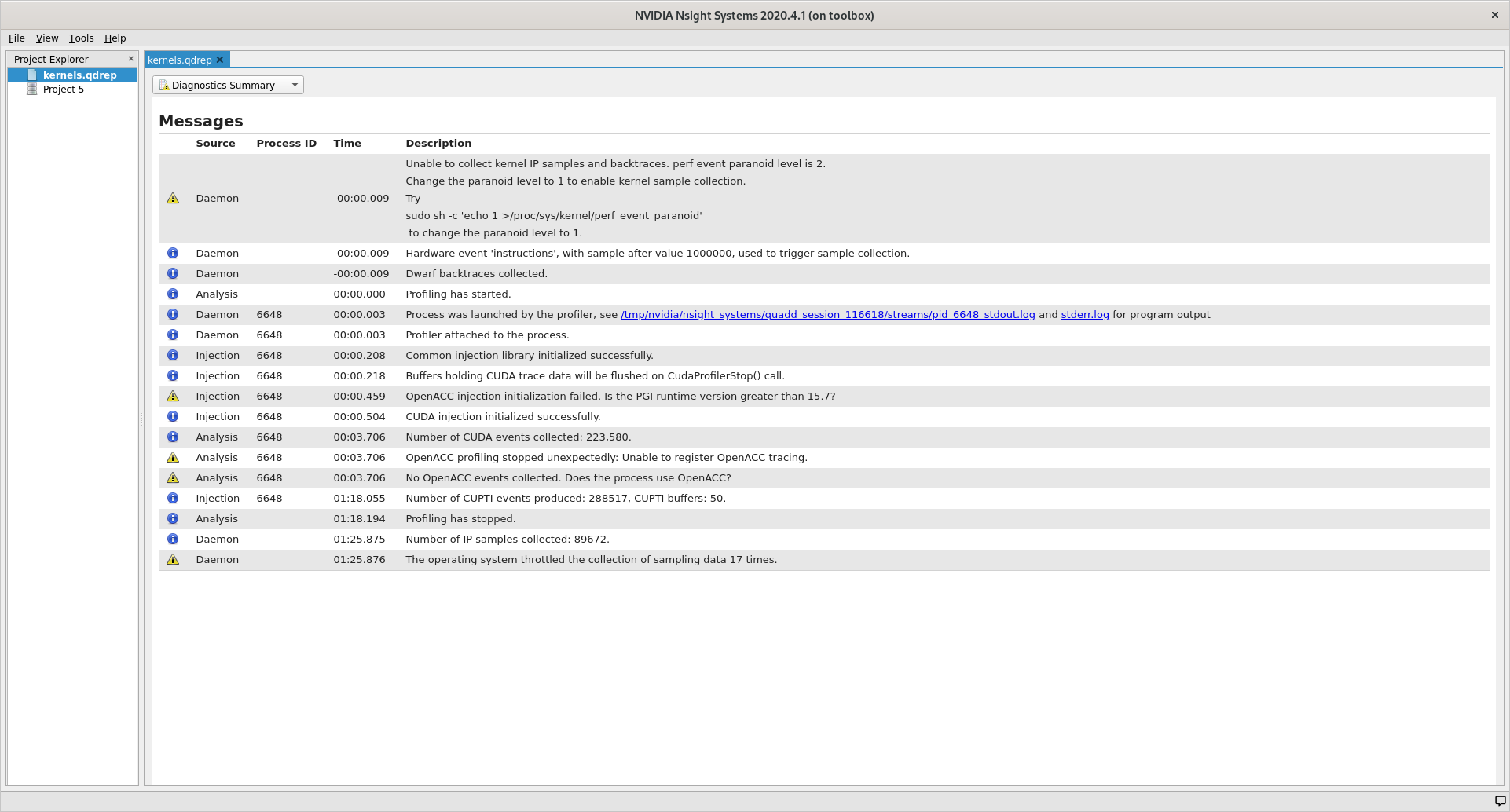After that the `Analysis Summary` give an overview of the profile. This view contains a lot of information which can be nice to review to ensure that the profile was configured correctly. Instances of good places to review are the `CLI command used` which shows how the profile was generated, `GPU info` which shows the accelerator in use and the `Analysis options` which show how `nsys` interpreted the command line arguments.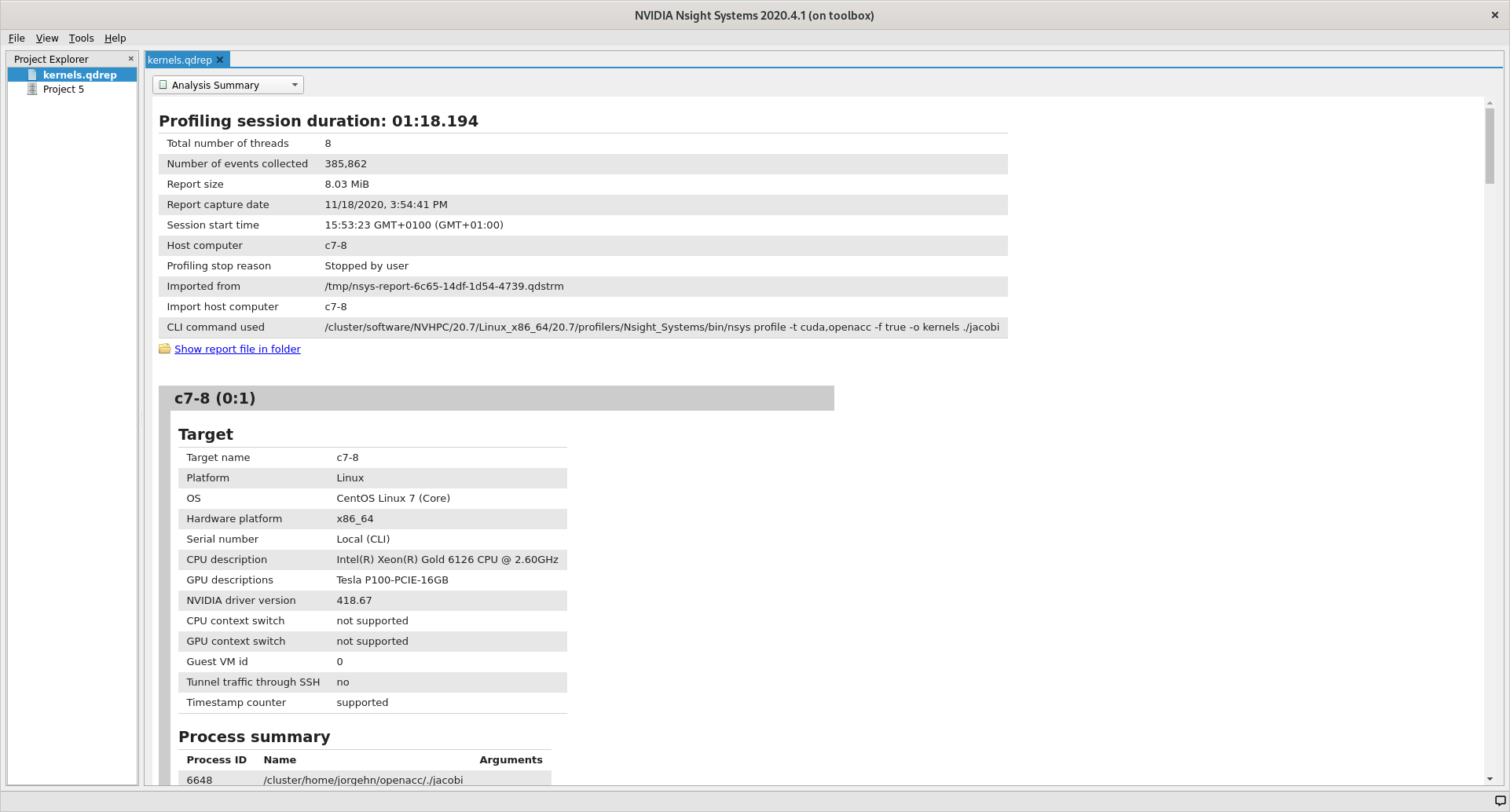The last view that we will detail here (because the two remaining are not that informative for understanding the profile information) is the `Timeline View`, which is the default view that we saw when we opened Nsight.

A good place to start with this view is the second dropdown, marked with `4`. In this dropdown we can select additional information to display about our profile results. By selecting one of the different `... View` options the profiler can show us which functions used what amount of the runtime in different ways. In the image below we have selected `Bottom-Up View` which sorts functions by placing the most time consuming ones at the top.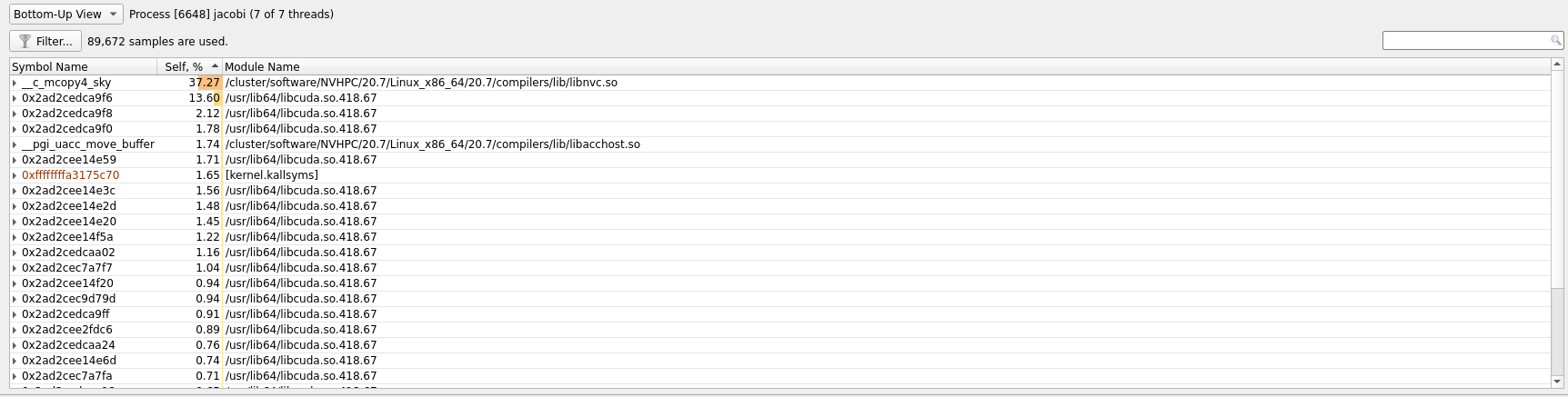In the timeline main view, we can see the usage of different APIs and the amount of CPU and GPU usage. A quick first thing to do is to click the arrow next to our GPU name so that it shows the percentage of `Kernels` usage and the percentage of `Memory` usage. In our current profile we can see that we are only using about `6%` of the `Kernels` resource which means that our GPU is spending only `6%` of its time actually doing useful compute.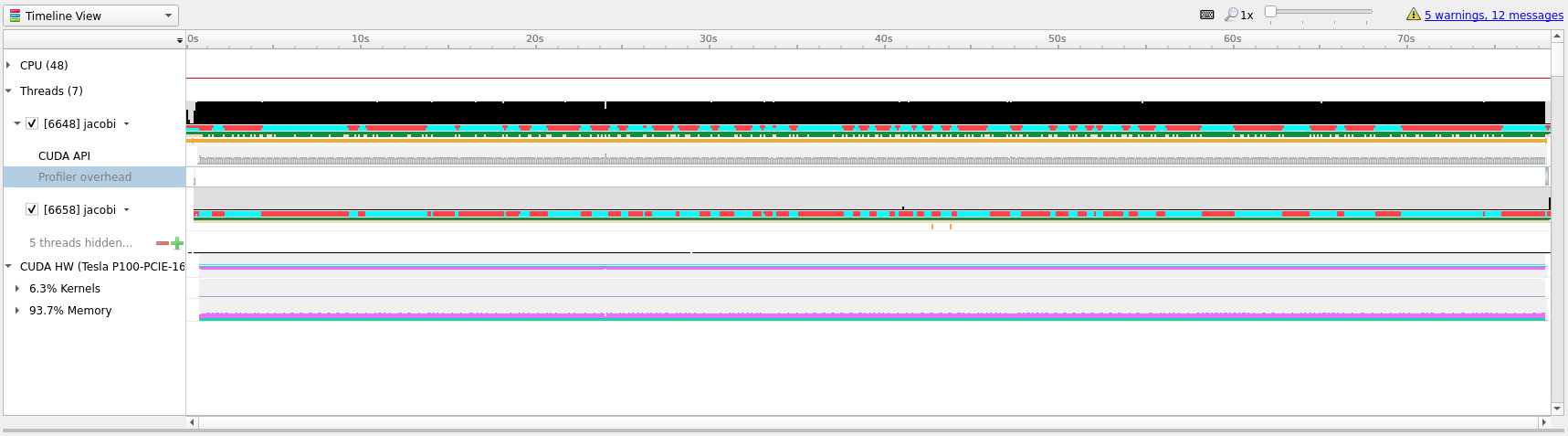To better understand what we are seeing in the timeline it is useful to zoom into specific areas to see what is going on. Use the mouse cursor to select a small column of the timeline area, right click and select `Zoom into selection`. Depending on how long the profile ran for it can be necessary doing this several times. Below we have tried to illustrate how far we would usually zoom in.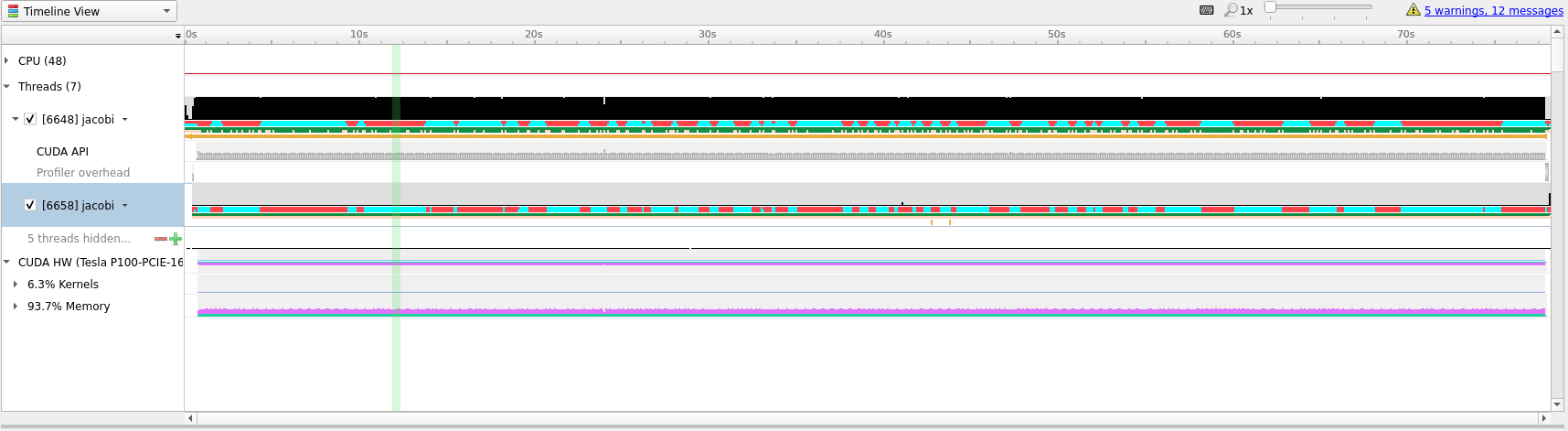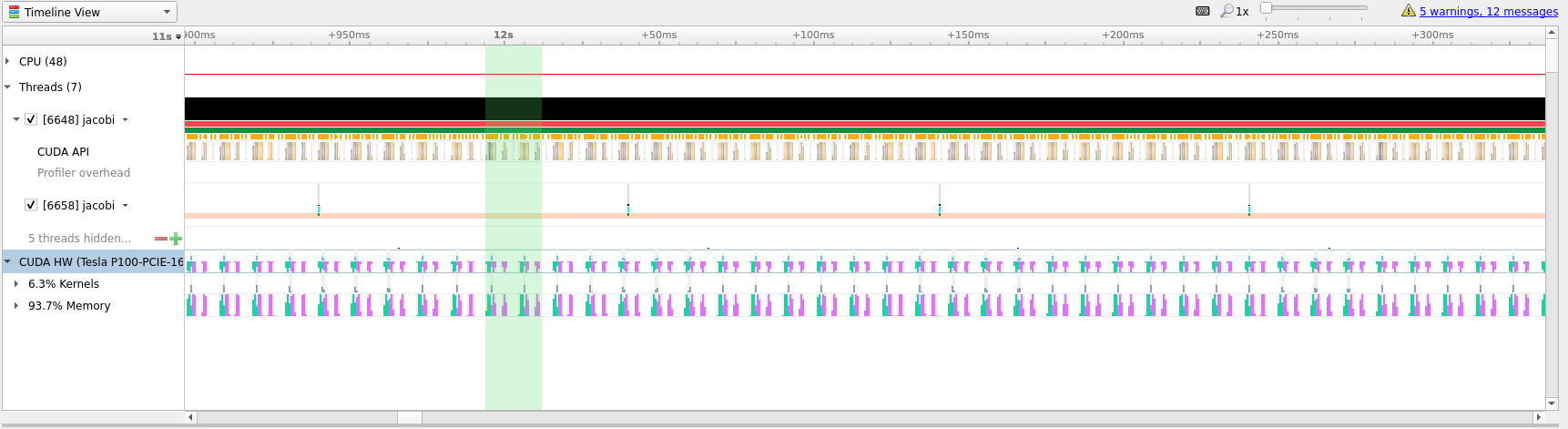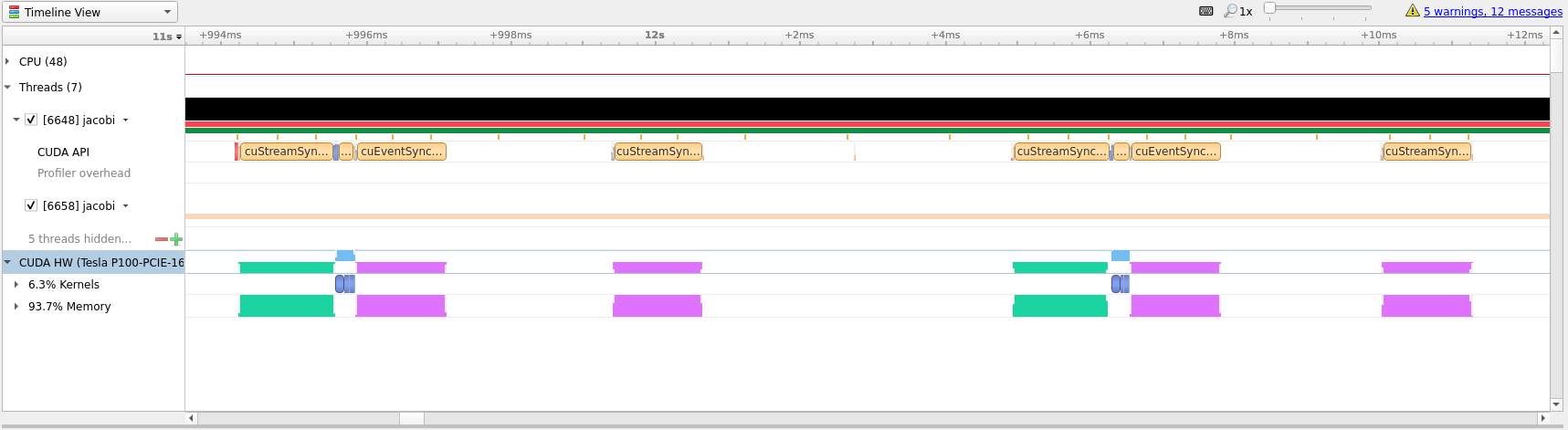In the last picture above we have zoomed in on what appears to be a cycle of two kernel launches. Remembering our code, that is most likely two iterations of the `while` loop where we placed our `kernels` directive inside.

## Profile Guided Optimization

Even though we have translated our program to run on the GPU it has not yet given us the results that we were after. Running on GPU resulted in a computation that is about `1.5` times slower than just running on CPU, but we can do better.

Looking at the zoomed in view of the timeline, in the image below, we can see that most of the time is taken up with transferring data between the CPU and the GPU.Optimizing data transfer is a crucial part of translating code to the GPU and accounts for most of the time spent optimizing a program for the GPU.

Looking at our `while` loop we can see that we are only interested in the final result after the loop exits which means that we should try to keep the data on the GPU and only transfer in and out at the beginning and end of the loop. To do this we will introduce the `#pragma acc data` clause which tells the compiler that we only want to do data movement for a given scope. The changes needed center around the `while` loop shown below.

```  #pragma acc data copy(array, arr_new)
while (error > MAX_ERROR && iterations < MAX_ITER) {
error = 0.;
#pragma acc kernels
{
// For each element take the average of the surrounding elements
for (int i = 1; i < NUM_ELEMENTS - 1; i++) {
for (int j = 1; j < NUM_ELEMENTS - 1; j++) {
arr_new[i][j] = 0.25 * (array[i][j + 1] +
array[i][j - 1] +
array[i - 1][j] +
array[i + 1][j]);
error = fmaxf (error, fabsf (arr_new[i][j] - array[i][j]));
}
}
// Transfer new array to old
for (int i = 1; i < NUM_ELEMENTS - 1; i++) {
for (int j = 1; j < NUM_ELEMENTS - 1; j++) {
array[i][j] = arr_new[i][j];
}
}
}
iterations += 1;
}
```

`jacobi_data.c`

Let us compile this on Saga and see if this results in better performance. Compile and run with the following commands.

```# Remember 'module load NVHPC/20.7' when logging in and out
\$ nvc -g -fast -acc -Minfo=accel -o jacobi jacobi_data.c
\$ sbatch kernels.job
```

Below we have included the timeline view of the updated profile.

`data.qdrep`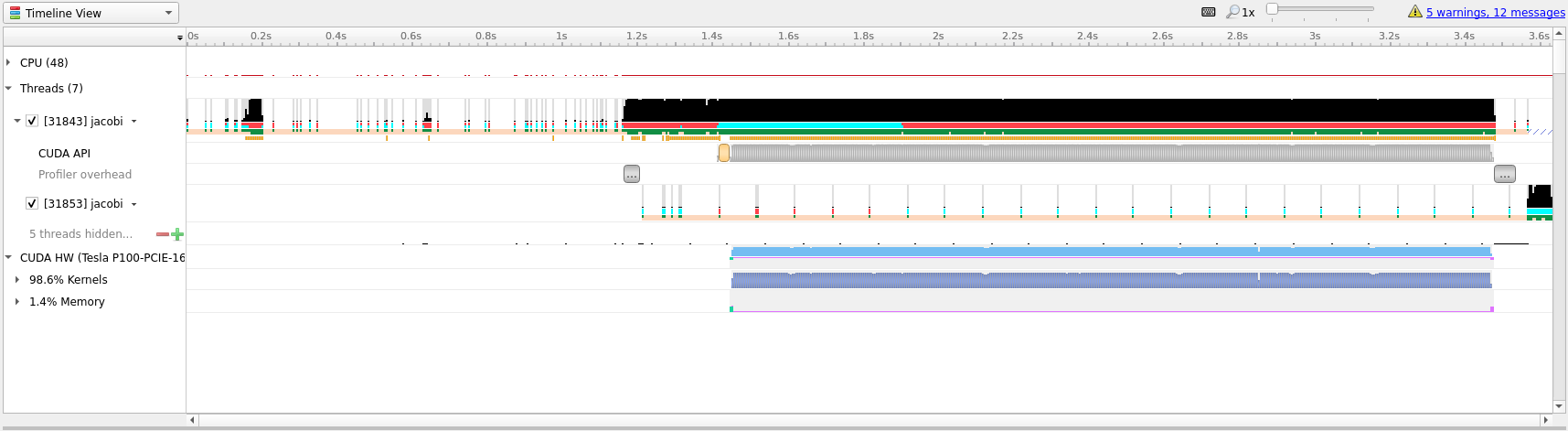Although this doesn’t look all that different from the previous profiles, notice that the timeline only goes to about `3.6` seconds, the previous profile went to above `70` seconds. Almost a `20x` speedup! Compared to our runs on the CPU this translation to the GPU has given us about a `10x` speedup. This shows the importance of data movement and is a good illustration of the optimization process, initially the code ran much slower on the GPU than on the CPU before becoming better than the CPU.

Doing better than this will be difficult, however, to introduce a few more concept that can be nice - we will perform a few more iterations on the code. However, do not expect great improvements.

The first improvement that we can do is to realize that `arr_new` will never be needed and is simply a scratch array for our computation, we can thus change our data directive to `#pragma acc data copy(array) create(arr_new)`. This tells the compiler that it should copy `array` from the CPU to the GPU when entering the loop and copy the data back from the GPU to CPU when exiting the scope. The `create(arr_new)` tells the compiler to only create the data on the GPU, it will not copy anything in or out, which is ok for us since we will overwrite it on first loop anyway and never use it after the loop.

The above optimization will net us very little so lets do some more. Instead of using the `kernels` directive we can take more control of the translation and tell the compiler that we would like to parallelize both loops. This is done with the `#pragma acc parallel loop` directive. Since we also want to do a reduction across all loops we can also add a reduction by writing `#pragma acc parallel loop reduction(max:error)` to the first loop. Lastly, we will apply the `collapse(n)` clause to both loop directives so that the compiler can combine the two loops into one large one, with the effect of exposing more parallelism for the GPU. The new code is show below.

```  #pragma acc data copy(array) create(arr_new)
while (error > MAX_ERROR && iterations < MAX_ITER) {
error = 0.;
#pragma acc parallel loop reduction(max:error) collapse(2)
for (int i = 1; i < NUM_ELEMENTS - 1; i++) {
for (int j = 1; j < NUM_ELEMENTS - 1; j++) {
arr_new[i][j] = 0.25 * (array[i][j + 1] +
array[i][j - 1] +
array[i - 1][j] +
array[i + 1][j]);
error = fmaxf (error, fabsf (arr_new[i][j] - array[i][j]));
}
}
#pragma acc parallel loop collapse(2)
for (int i = 1; i < NUM_ELEMENTS - 1; i++) {
for (int j = 1; j < NUM_ELEMENTS - 1; j++) {
array[i][j] = arr_new[i][j];
}
}
iterations += 1;
}
```

`jacobi_optimized.c`

Looking at the generated profile, `optimized.qdrep` shown below, we can see that we managed to eek out slightly more performance, but not that much.

`optimized.qdrep`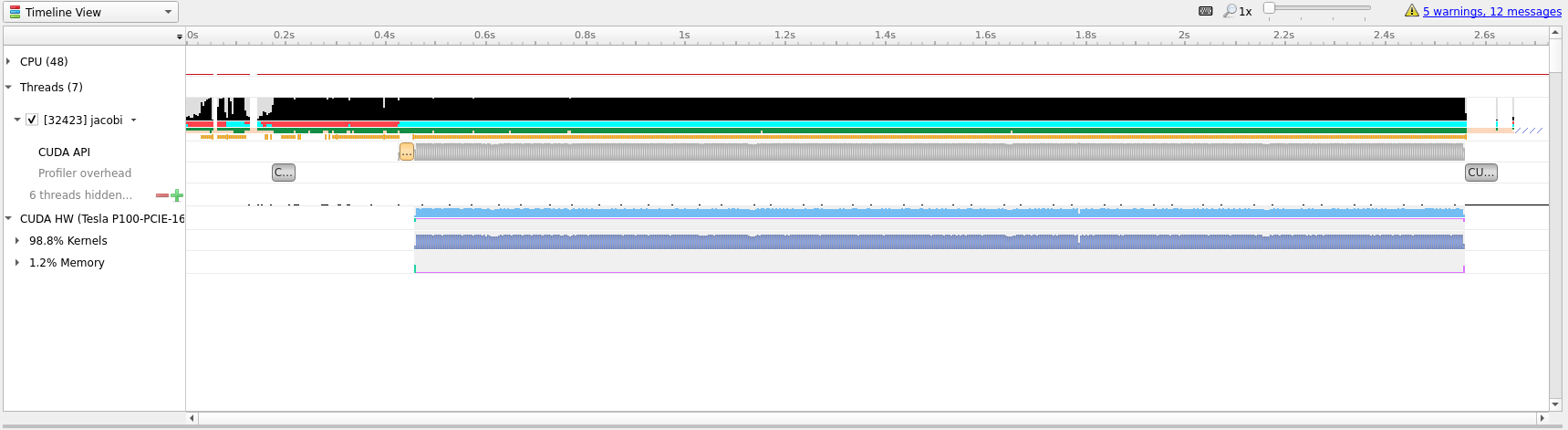Compared to the initial translation we can see now that the ratio of `Kernels` to `Memory` on the GPU is much better, `98%` spent actually doing useful compute.

If we zoom in, as in the image below, we can see that there is not much wasted time between useful compute. Going further with OpenACC is most likely not that useful and getting this to run even quicker will likely require a rewrite to `CUDA` which is outside the scope and intention of this guide.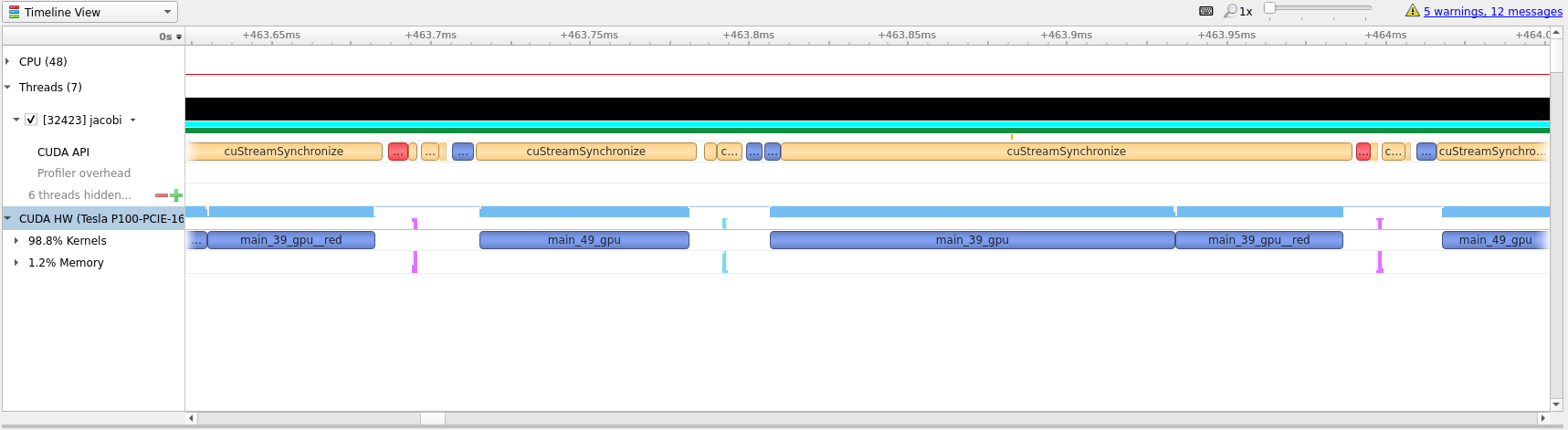One way to see how well we have optimized the code is to look at the white space between compute regions. In our initial translation these white spaces lasted for around `4` milliseconds. In the optimized profile the whitespace between kernels amount to around `32` microseconds.

## Summary

In this guide we have shown how to use OpenACC to transition a simple `C` example from running on the CPU to running the main calculations on GPU. We have detailed how such code can be used, compiled and run on Saga. And, we have introduced Nsight and how it can be used to profile and guide OpenACC transitions.

## Tips

• Do not expect miracles! Translating a large code base to run on GPU is a large undertaking and should not be taken lightly. Just getting a large code base to run on GPU and having almost the same performance as the CPU code is extremely good! Optimizing for GPUs require time and patience.

• Always start with the `kernels` directive and study the compiler output. This should guide your next steps. The information outputted by the compile will usually tell you if the scope of the directive can be run effectively on GPU and if you should take some steps to rewrite parts of the code.

Compiler output like `Loop carried dependence of <name> prevents   parallelization` and `Loop carried backward dependence of <name> prevents   vectorization` are clear indications that the compiler is not able to automatically translate the code and a rewrite might be necessary.

• Data movement is paramount. If you know some data is only needed to read from use `copyin`, `copyout` if it is only written to, `present` can be nice if you know the data should already be present on the GPU and `copy` ensures that the program functions as expected. OpenACC has several directive that can be used to perform data management and some are even scoped for the entire program.

• Be structured in your approach. Only translate one scope at a time. This ensures that you can focus on a small area and get less compiler output to study. Profiling between each round may not be necessary, but it can be valuable to know what is happening.

## Fortran

As mentioned in the beginning of this document, OpenACC also supports `Fortran`. Directives in Fortran can be added in a similar fashion to OpenMP directives, with `!\$acc` instead of `!\$OMP`. Below is an example of matrix multiplication with the `!\$acc kernels` directive.

```program mxm
integer, parameter :: r8  = selected_real_kind(p=15,r=307)
parameter(N=4000)
real(r8) a(N,N), b(N,N) , c(N,N), temp
integer i, j, l, c1, c2

call random_number(a)
call random_number(b)

call system_clock(count=c1)

!\$acc kernels
do j = 1,N
do l = 1,N
do i = 1,N
c(i,j) = c(i,j) + a(i,l)*b(l,j)
enddo
enddo
enddo
!\$acc end kernels
call system_clock(count=c2)

write(*,*) "Calc time : ",(c2-c1)/1e6," secs"
write(*,*) c(1,1), c(N,N), sum(c)
end program mxm
```

`mxm.f90`

On Saga, load the `NVHPC/20.7` module and compile with `nvfortran` as follows:

```\$ module load NVHPC/20.7
\$ nvfortran -o mxm -fast -acc -gpu=cc60 -Minfo=accel mxm.f90
```

To run the program on Saga with GPUs use:

```\$ srun --account=<your project number> --time=02:00 --mem-per-cpu=512M --partition=accel --gpus=1 ./mxm
```

This program is as close to the best case scenario possible for accelerators and, on Saga, gives a speedup of `24x` compared to a single CPU core.

Flags

Run time

Speedup

`-fast` (single CPU core)

48.9 seconds

1 x

`-fast -acc -gpu=cc60`

2.0 seconds

24 x

You can profile `Fortran` programs in the same way you would for `C/C++`, using `nsys profile` and the flag `-t cuda,openacc`.